Mean curvature

of a surface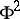in-dimensional Euclidean space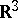Half of the sum of the principal curvatures (cf. Principal curvature)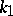and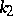, calculated at a pointof this surface: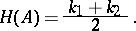For a hypersurface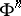in the Euclidean space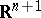, this formula is generalized in the following way: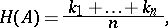where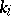,, are the principal curvatures of the hypersurface, calculated at a point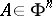.

The mean curvature of a surface incan be expressed by means of the coefficients of the first and second fundamental forms of this surface:where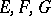are the coefficients of the first fundamental form, andare the coefficients of the second fundamental form, calculated at a point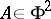. In the particular case where the surface is defined by an equation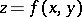, the mean curvature is calculated using the formula: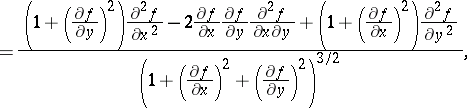which is generalized for a hypersurfacein, defined by the equation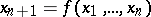, as follows:where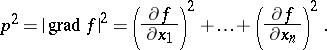For an-dimensional submanifoldof an-dimensional Euclidean space of codimension, the mean curvature generalizes to the notion of the mean curvature normal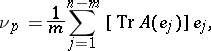where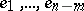is an orthonormal frame of the normal space (cf. Normal space (to a surface)) ofatand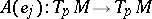(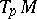denotes the tangent space toat) is the shape operator ofatin the direction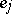, which is related to the second fundamental tensorofatby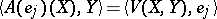.